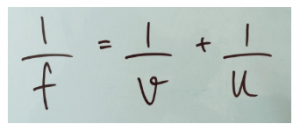# Thin Lens Formula for Concave and Convex Lenses

## Thin Lens Formula with Magnification for Concave and Convex Lenses

First of all, what is a thin lens? If the thickness of a lens is negligible in comparison to the radius of curvature, it is a thin lens. The following typical equation is used to determine a relation between the focal length of the lens, the distance of object and distance of image.where,

$f$ is the focal length

$v$ is the distance of the image from the optical centre

$u$ is the distance of the object from the optical centre

If the focal length is negative, it implies that the lens is concave (diverging), while if positive it implies that the lens is convex (converging lenses). If the image distance is calculated to be negative, it implies that the image formed is virtual and on the same side as the object.

The magnification ($M$) of the image formed can be calculated using the following formula.where,

$v$ is the object height

$u$ is the image height

If ‘$i$’ is positive, the image is upright and if ‘$i$’ is negative, the image is inverted.

The two formulas given above are together referred to as thin lens formula. You can try the following sample problem using this.

A 4 cm tall candle is placed in front of a double convex lens. The lens has a focal length of 15.2 cm. The object has been placed 45.7 cm from the vertex. Where will the image be formed and what will its size be?

Ans: Image distance = 22.8cm; Image Height = -1.99cm

Solve application questions using thin lens formula by joining other students here at BYJU’s.